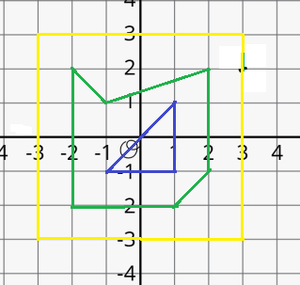Find number of Polygons lying inside each given Polygons on Cartesian Plane

• Last Updated : 24 Dec, 2021

Given N non-intersecting nested polygons, and a 2D array arr[][] of pairs, where each cell of the array represents the coordinates of the vertices of a polygon. The task is to find the count of polygons lying inside each polygon.

Examples:

Input: N = 3, arr[][][] = {{{-2, 2}, {-1, 1}, {2, 2}, {2, -1}, {1, -2}, {-2, -2}}, {{-1, -1}, {1, -1}, {1, 1}}, {{3, 3}, {-3, 3}, {-3, -3}, {3, -3}}}
Output: 1 0 2
Explanation:1. The polygon represented at index 0, is the green-colored polygon shown in the picture above.
2. The polygon represented at index 1, is the blue-colored polygon shown in the picture above.
3. The polygon represented at index 2, is the yellow-colored polygon shown in the picture above.

Therefore, there is 1 polygon inside the polygon at index 0, and 0 polygons inside the polygon at index 1, and 2 polygons inside the polygon at index 2.

Recommended: Please try your approach on {IDE} first, before moving on to the solution.

Approach: The above problem can be solved based on the following observations:

1. It can be observed that the polygon lying outside will have the largest X- coordinates. The maximum X-coordinates of the polygon will decrease, as one will go towards the inside, as it is given that polygons are nested and non-intersecting.
2. Therefore, sorting the polygons by the maximum X-coordinate will give the correct order of the polygons lying.

Follow the steps below to solve the problem:

• Initialize a 2D array say maximumX[][] of size N*2 to store the pair of maximum X-coordinates of a polygon and the index value of the polygon.
• Iterate over the array arr[][] using the variable, i perform the following steps:
• Initialize a variable, say maxX, to store the maximum X-coordinate of the current polygon.
• Iterate over the array arr[i] using the variable j and in each iteration update the maxX as maxX = max(maxX, arr[i][j]).
• Assign the pair {maxX, i} to maximumX[i].
• Sort the array of pairs in descending order by the first element using a custom comparator.
• Initialize an array, say res[], to store the count of polygons lying inside the current polygon.
• Iterate over the range [0, N-1] using the variable i and in each iteration assign N-i-1 to res[maximumX[i]], as there are N-i-1 polygons lying inside the maximumX[i]th polygon.
• Finally, after completing the above steps, print the array res[] as the answer.

Below is the implementation of the above approach:

Java

 // Java program for above approachimport java.util.*;  class GFG {      // Function to sort a 2D    // array using custom a comparator    public static void sort2d(int[][] arr)    {        Arrays.sort(arr, new Comparator() {            public int compare(final int[] a,                               final int[] b)            {                if (a < b)                    return 1;                else                    return -1;            }        });    }      // Function to calculate maximum sum    static void findAllPolygons(int N,                                int[][][] arr)    {        // Stores the maximum X co-        // ordinate of every polygon        int[][] maximumX = new int[N];          // Traverse the array arr[][]        for (int i = 0; i < N; i++) {              // Stores the max X co-            // ordinate of current            // polygon            int maxX = Integer.MIN_VALUE;              // Traverse over the vertices            // of the current polygon              for (int j = 0; j < arr[i].length; j++) {                // Update maxX                maxX = Math.max(maxX, arr[i][j]);            }              // Assign maxX to maximumX[i]            maximumX[i] = maxX;              // Assign i to maximumX[i]            maximumX[i] = i;        }          // Sort the array of pairs        // maximumX in descending        // order by first element        sort2d(maximumX);          // Stores the count of        // polygons lying inside        // a polygon        int[] res = new int[N];          // Traverse the maximumX array        for (int i = 0; i < N; i++) {            // Update value at            // maximumX[i] in            // res            res[maximumX[i]] = N - 1 - i;        }          // Print the array array res        for (int i = 0; i < N; i++) {            System.out.print(res[i] + " ");        }    }      // Driver Code    public static void main(String[] args)    {          int N = 3;        int[][][] arr = {            { { -2, 2 }, { -1, 1 }, { 2, 2 }, { 2, -1 }, { 1, -2 }, { -2, -2 } },            { { -1, -1 }, { 1, -1 }, { 1, 1 } },            { { 3, 3 }, { -3, 3 }, { -3, -3 }, { 3, -3 } }        };          findAllPolygons(N, arr);    }}
Output
1 0 2

Time Complexity: O(M + N log N), Where M maximum size of a polygon
Auxiliary Space: O(N)

My Personal Notes arrow_drop_up